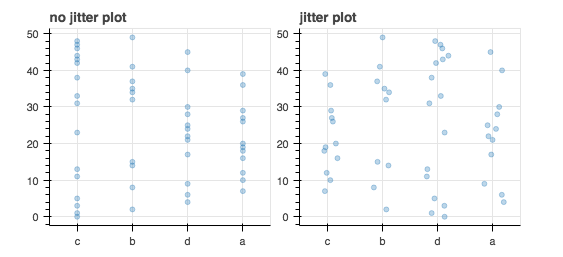# learning to use Jitter()

Can anyone help me understand how to use the new Jitter functionality for real categorical plots?
The only example I can find is at http://bokeh.pydata.org/en/latest/docs/gallery/jitter.html

That’s a toy example that hardcodes the categories along the x-axis.

``````p.circle(x={'value': 1, 'transform': Jitter(width=0.4)}, y=y1,
color="navy", alpha=0.3)
``````

What does it mean to pass a dictionary for ‘x’?

What are the other keys that can be defined in that dict?

The documentation says you can pass a DataSpecProperty, but that word is not defined.

If I try to mimic that example for real data, I have to deconstruct the plot into separate calls to fig.circle() for each categorical group.

As if that’s not bad enough, it still doesn’t really work because I can’t know which ‘value’ number maps to which category along the range of the x-axis.

So does anyone have a better example for Jitter?from bokeh.plotting import figure, show

from bokeh.layouts import row

from bokeh.models.sources import ColumnDataSource

from bokeh.models.transforms import Jitter

from bokeh.models import Jitter

import pandas as pd

import numpy as np

df = pd.DataFrame.from_dict({

“model” : np.random.choice([‘a’, ‘b’, ‘c’, ‘d’], 50),

“omega” : range(50)

})

factors = list(df.model.unique())

# without jitter.

nojitter = figure(width=250, plot_height=250, title=“no jitter plot”, x_range=factors)

nojitter.toolbar_location = None

nojitter.circle(x=“model”, y=“omega”, source=ColumnDataSource(data=df),

alpha=0.3, size=5)

# with jitter

jitter = figure(width=250, plot_height=250, title=“jitter plot”, x_range=factors)

jitter.toolbar_location = None

for i,(k,grp) in enumerate(df.groupby(“model”)):

jitter.circle(x={‘value’:i+1, ‘transform’: Jitter(width=0.4)}, y=“omega”,

source=ColumnDataSource(data=grp),

alpha=0.3, size=5)

p = row(nojitter, jitter)

show§

``

Hi chris,

Can anyone help me understand how to use the new Jitter functionality for real categorical plots?
The only example I can find is at http://bokeh.pydata.org/en/latest/docs/gallery/jitter.html
That's a toy example that hardcodes the categories along the x-axis.

p.circle(x={'value': 1, 'transform': Jitter(width=0.4)}, y=y1,

color="navy", alpha=0.3)

What does it mean to pass a dictionary for 'x'?
What are the other keys that can be defined in that dict?
The documentation says you can pass a DataSpecProperty, but that word is not defined.

Before computed transforms (e.g. Jitter), DataSpecs are something that have by and large been an implementation detail, and hidden from users. With this new capability, they probably merit some better documentation. But the gist is this: under the covers, 'x' is always a dictionary! In particular, x is a DataSpec, which is a dictionary that must have one of two fields:

{ 'field': 'some_CDS_colname' }

or

{ 'value': 10 }

There can sometimes be other keys (e.g. "units"). The main purpose of this is to map the visual characteristics of a specific glyph either to a fixed value, or to values from a ColumnDataSource column. This switching happens automatically in BokehJS. (The only real time this distinction has ever popped up before for users is with the value for Text glyphs --- the value is text, but so are column names, requiring some measure of explicitness to disambiguate intent).

But very recently a new contributor made a wonderful PR that added a new and powerful capability to Bokeh: the option to map not just a fixed value, or a column to glyph attributes, but to map a *transformed* fixed value or attribute (where the transformation happens in the browser). This is expressed as DataSpec, but with now a new possible key for the transform as you have seen:

{'value': 'mycol', 'transform': Jitter(width=0.4)}

On the BokehJS side this makes the values from 'mycol' be run through the Jitter function before they are used to draw, or whatever. So far the only "built in" example is Jitter. But Sarah Bird is working on a PR for ColorMapper transforms (no more columns of colors!) here:

And hopefully a CustomJSTransform will be coming soon too, to enable truly any sort of client side mappings (e.g. the sizes of a bubble chart derevid from a real data column in the browser).

If I try to mimic that example for real data, I have to deconstruct the plot into separate calls to fig.circle() for each categorical group.
As if that's not bad enough, it still doesn't really work because I can't know which 'value' number maps to which category along the range of the x-axis.

Constructive criticism are certainly welcome, but it's also good to try and ask questions and take the time to understand the context of things first as well. The central motive behind the bokeh.plotting interface is to attach data columns *directly* to visual attributes. So using bokeh.plotting always implies doing your own grouping. The higher level bokeh.charts API specifically to do things like grouping and aggregations on data, and to generate plots based on these higher level constructions. However, the computed transform capability (and Jitter in particular) is *very* new. A good idea would be to integrate this new capability into bokeh.charts so that a jittered chard it a one-liner. But the core team is stretched extremely thin right now, and is forcused on other important priorities. As this would be pure python development, it might make a nice PR for a new contributor to take on. (We are always happy to help new contributors.)

Thanks,

Bryan

···

So does anyone have a better example for Jitter?

from bokeh.plotting import figure, show
from bokeh.layouts import row
from bokeh.models.sources import ColumnDataSource
from bokeh.models.transforms import Jitter
from bokeh.models import Jitter
import pandas as pd
import numpy as np

df = pd.DataFrame.from_dict({
"model" : np.random.choice(['a', 'b', 'c', 'd'], 50),
"omega" : range(50)
})
factors = list(df.model.unique())

# without jitter.
nojitter = figure(width=250, plot_height=250, title="no jitter plot", x_range=factors)
nojitter.toolbar_location = None
nojitter.circle(x="model", y="omega", source=ColumnDataSource(data=df),
alpha=0.3, size=5)

# with jitter
jitter = figure(width=250, plot_height=250, title="jitter plot", x_range=factors)
jitter.toolbar_location = None
for i,(k,grp) in enumerate(df.groupby("model")):
jitter.circle(x={'value':i+1, 'transform': Jitter(width=0.4)}, y="omega",
source=ColumnDataSource(data=grp),
alpha=0.3, size=5)

p = row(nojitter, jitter)

show(p)

--
You received this message because you are subscribed to the Google Groups "Bokeh Discussion - Public" group.
To unsubscribe from this group and stop receiving emails from it, send an email to [email protected].
To post to this group, send email to [email protected].
To view this discussion on the web visit https://groups.google.com/a/continuum.io/d/msgid/bokeh/c6977d5a-35cb-4e3d-86eb-bfcabba3513c%40continuum.io.